# Queues Definition of a Queue Examples of Queues

• Slides: 21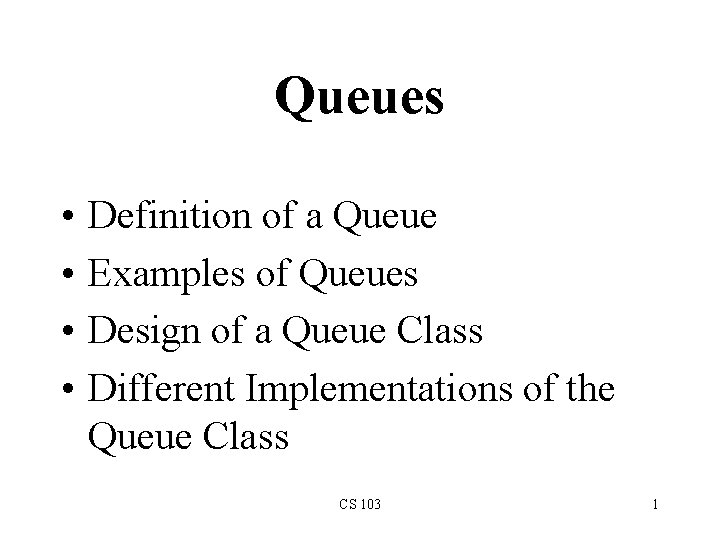Queues • • Definition of a Queue Examples of Queues Design of a Queue Class Different Implementations of the Queue Class CS 103 1Definition of a Queue • A queue is a data structure that models/enforces the first-come first-serve order, or equivalently the first-in first-out (FIFO) order. • That is, the element that is inserted first into the queue will be the element that will deleted first, and the element that is inserted last is deleted last. • A waiting line is a good real-life example of a queue. (In fact, the Britich word for “line” is “queue”. ) CS 103 2A Graphic Model of a Queue Head: All items are deleted from this end Tail: All new items are added on this end CS 103 3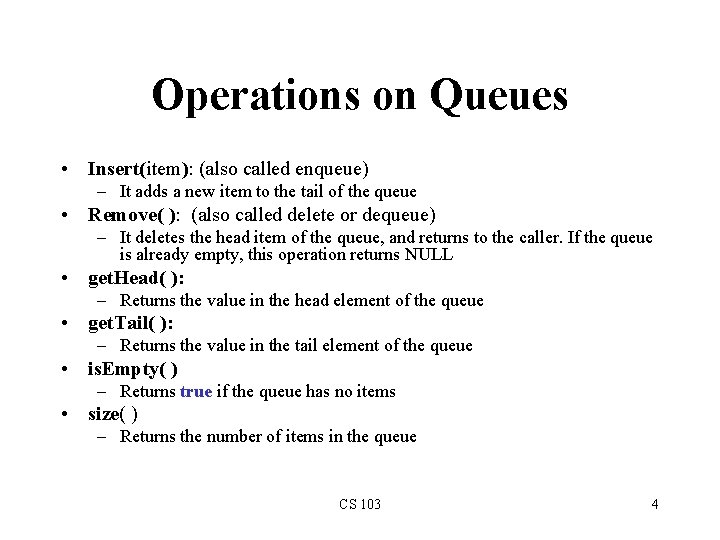Operations on Queues • Insert(item): (also called enqueue) – It adds a new item to the tail of the queue • Remove( ): (also called delete or dequeue) – It deletes the head item of the queue, and returns to the caller. If the queue is already empty, this operation returns NULL • get. Head( ): – Returns the value in the head element of the queue • get. Tail( ): – Returns the value in the tail element of the queue • is. Empty( ) – Returns true if the queue has no items • size( ) – Returns the number of items in the queue CS 103 4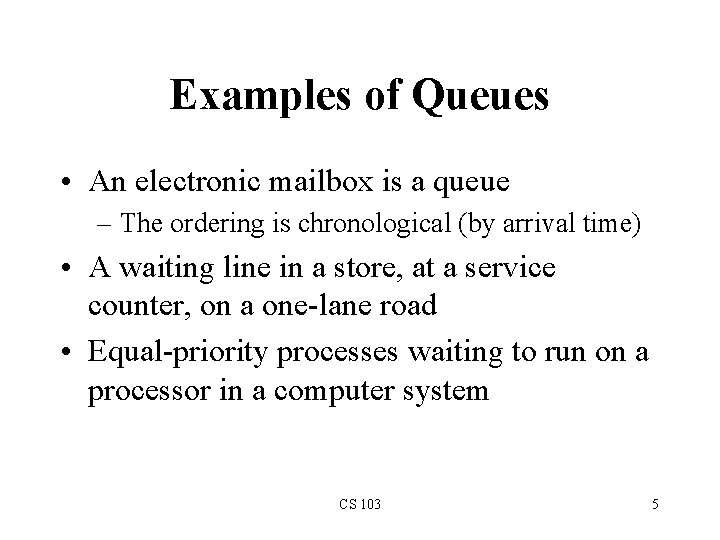Examples of Queues • An electronic mailbox is a queue – The ordering is chronological (by arrival time) • A waiting line in a store, at a service counter, on a one-lane road • Equal-priority processes waiting to run on a processor in a computer system CS 103 5Queue as a Class • Much like stacks and linked lists were designed and implemented as classes, a queue can be conveniently packaged as a class • It seems natural to think of a queue as similar to a linked list, but with more basic operations, to enforce the FIFO order of insertion and deletion CS 103 6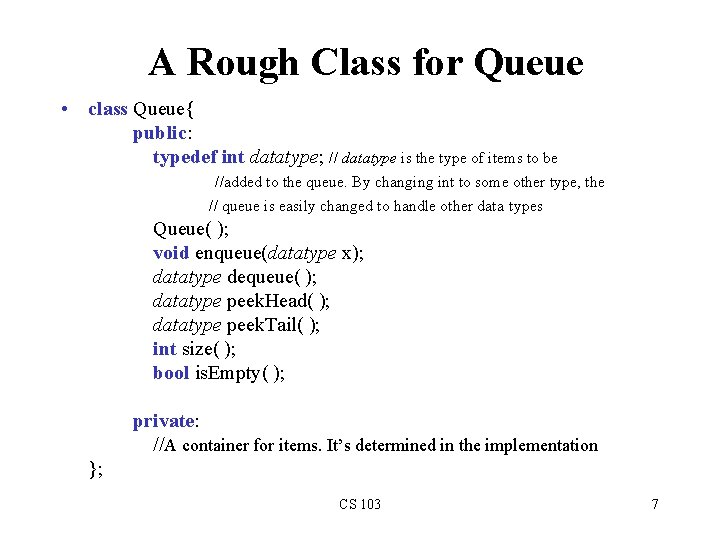A Rough Class for Queue • class Queue{ public: typedef int datatype; // datatype is the type of items to be //added to the queue. By changing int to some other type, the // queue is easily changed to handle other data types Queue( ); void enqueue(datatype x); datatype dequeue( ); datatype peek. Head( ); datatype peek. Tail( ); int size( ); bool is. Empty( ); private: //A container for items. It’s determined in the implementation }; CS 103 7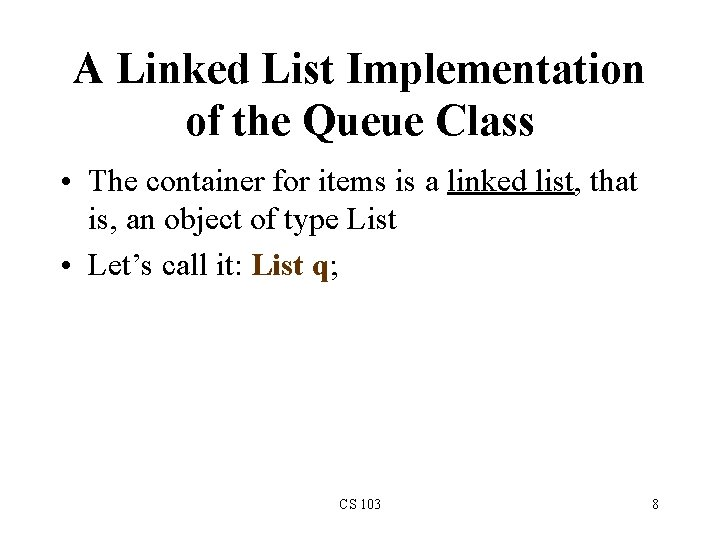A Linked List Implementation of the Queue Class • The container for items is a linked list, that is, an object of type List • Let’s call it: List q; CS 103 8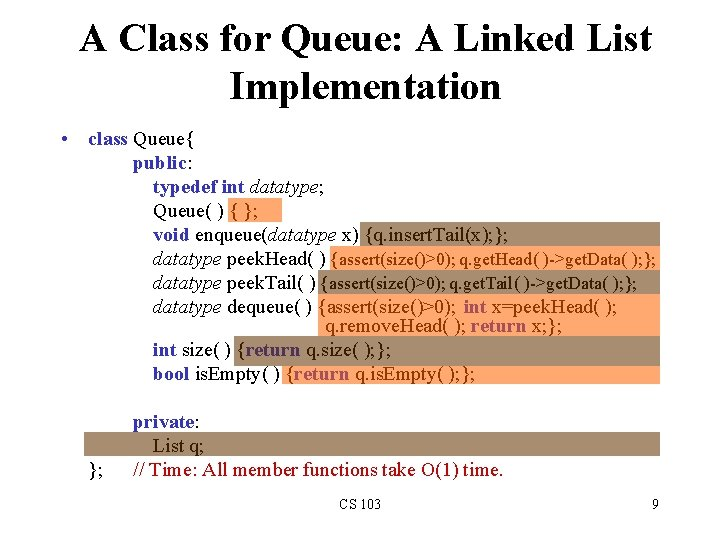A Class for Queue: A Linked List Implementation • class Queue{ public: typedef int datatype; Queue( ) { }; void enqueue(datatype x) {q. insert. Tail(x); }; datatype peek. Head( ) {assert(size()>0); q. get. Head( )->get. Data( ); }; datatype peek. Tail( ) {assert(size()>0); q. get. Tail( )->get. Data( ); }; datatype dequeue( ) {assert(size()>0); int x=peek. Head( ); q. remove. Head( ); return x; }; int size( ) {return q. size( ); }; bool is. Empty( ) {return q. is. Empty( ); }; private: List q; // Time: All member functions take O(1) time. CS 103 9A Dynamic-Array Implementation of the Queue Class • The container for items is a dynamic array • It is accomplished as: datatype *p=new datatype; CS 103 10A Class for Queue using Dynamic Arrays • class Queue{ public: typedef int datatype; Queue(int capacity = 50) ; void enqueue(datatype x); datatype peek. Head( ); int size( ); datatype dequeue( ); datatype peek. Tail( ); bool is. Empty( ); private: int capacity; // default value is 50 datatype *p; // pointer a dynamic array created by constructor int length; // number of actual elements in the queue int head; // index of the head element in the array int tail; // index of the tail element in the array }; CS 103 11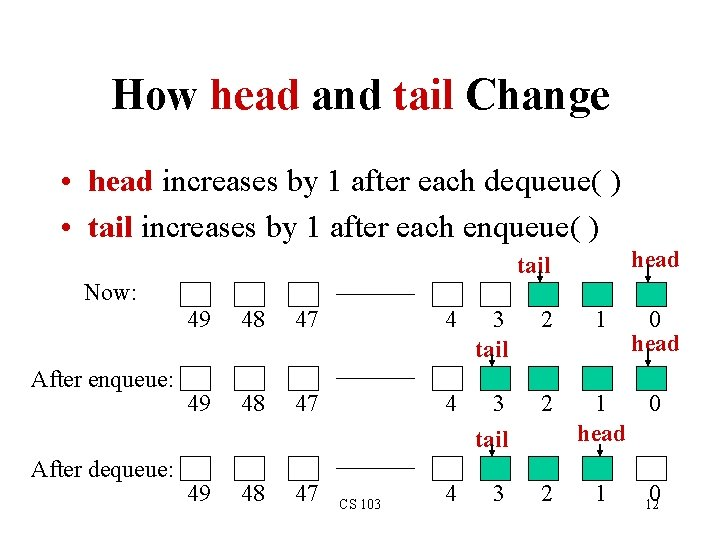How head and tail Change • head increases by 1 after each dequeue( ) • tail increases by 1 after each enqueue( ) head tail Now: After enqueue: After dequeue: 49 48 47 4 3 tail 2 49 48 47 4 3 2 CS 103 1 0 head 1 0 12False-Overflow Issue First • Suppose 50 calls to enqueue have been made, so now the queue array is full 49 48 47 4 3 2 1 0 • Assume 4 calls to dequeue( ) are made 49 48 47 4 3 2 1 0 • Assume a call to enqueue( ) is made now. The tail part seems to have no space, but the front has 4 unused spaces; if never used, they are wasted. CS 103 13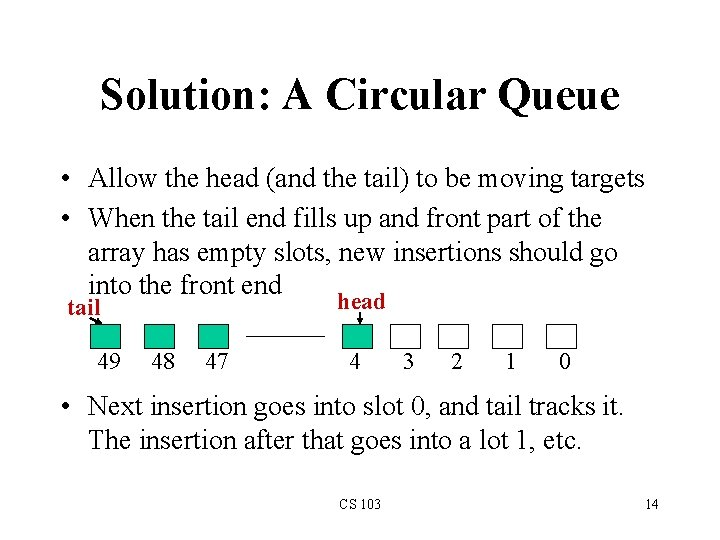Solution: A Circular Queue • Allow the head (and the tail) to be moving targets • When the tail end fills up and front part of the array has empty slots, new insertions should go into the front end head tail 49 48 47 4 3 2 1 0 • Next insertion goes into slot 0, and tail tracks it. The insertion after that goes into a lot 1, etc. CS 103 14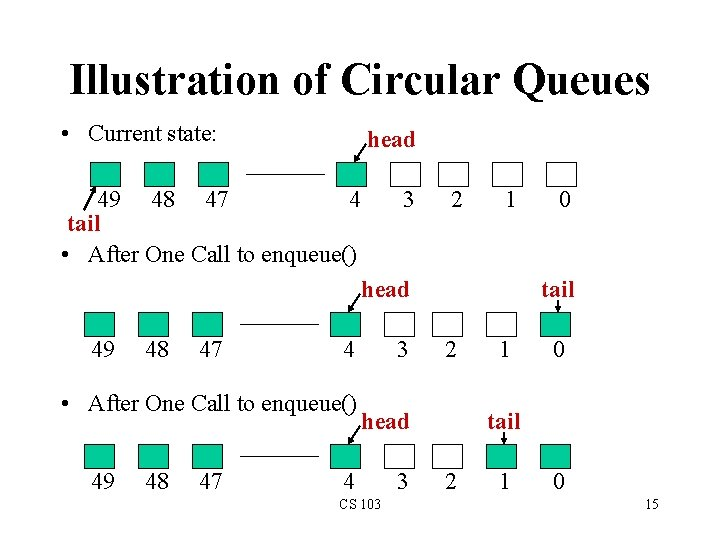Illustration of Circular Queues • Current state: head 4 49 48 47 tail • After One Call to enqueue() 3 2 1 head 49 48 47 4 • After One Call to enqueue() 49 48 47 3 tail 2 head 4 CS 103 3 0 1 0 tail 2 1 0 15Numerics for Circular Queues • head increases by (1 modulo capacity) after each dequeue( ): head = (head +1) % capacity; • tail increases by (1 modulo capacity) after each enqueue( ): tail = (tail +1) % capacity; CS 103 16Complete Implementation of Queues with Dynamic Arrays • Show the class structure as a refresher (next slide) • The implementations of the constructor and member functions will follow afterwards CS 103 17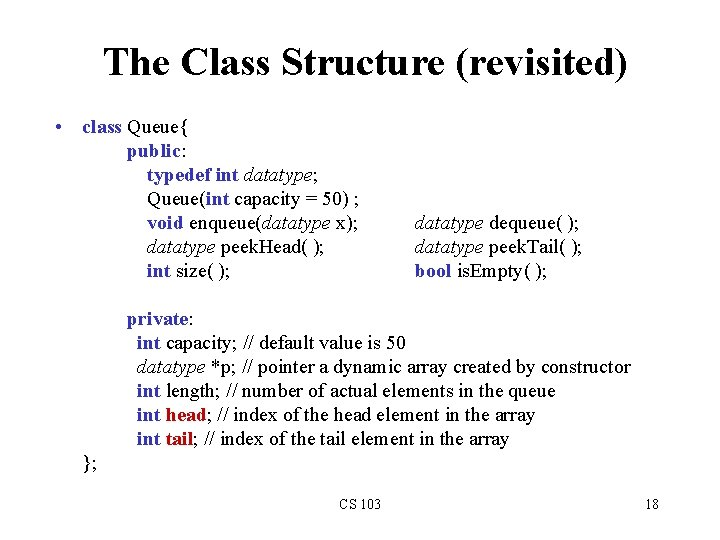The Class Structure (revisited) • class Queue{ public: typedef int datatype; Queue(int capacity = 50) ; void enqueue(datatype x); datatype peek. Head( ); int size( ); datatype dequeue( ); datatype peek. Tail( ); bool is. Empty( ); private: int capacity; // default value is 50 datatype *p; // pointer a dynamic array created by constructor int length; // number of actual elements in the queue int head; // index of the head element in the array int tail; // index of the tail element in the array }; CS 103 18Implementations of the Members Queue: : Queue(int capacity){ this. capacity = capacity; p = new datatype[capacity]; length=0; head=0; tail=0; }; int Queue: : size( ){return length; }; bool Queue: : is. Empty( ) {return (length==0); }; Queue: : datatype Queue: : peek. Head( ){assert(length>0); return p[head]; }; Queue: : datatype Queue: : peek. Tail( ){assert(length>0); return p[tail]; }; Time: All O(1) time. CS 103 19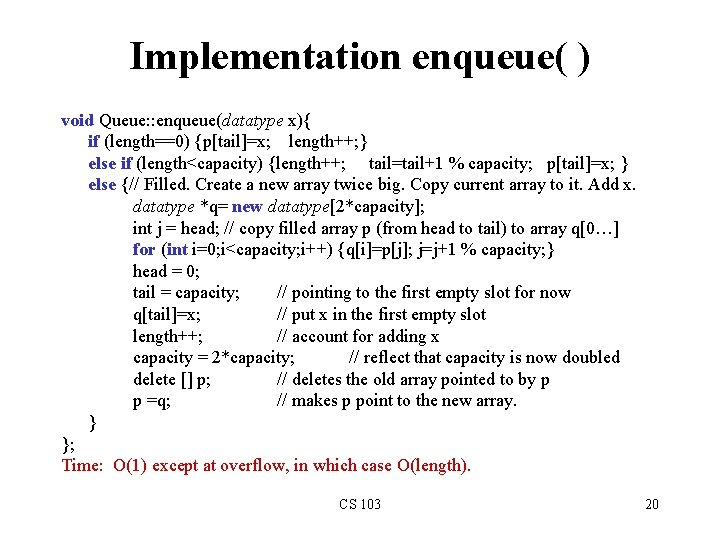Implementation enqueue( ) void Queue: : enqueue(datatype x){ if (length==0) {p[tail]=x; length++; } else if (length<capacity) {length++; tail=tail+1 % capacity; p[tail]=x; } else {// Filled. Create a new array twice big. Copy current array to it. Add x. datatype *q= new datatype[2*capacity]; int j = head; // copy filled array p (from head to tail) to array q[0…] for (int i=0; i<capacity; i++) {q[i]=p[j]; j=j+1 % capacity; } head = 0; tail = capacity; // pointing to the first empty slot for now q[tail]=x; // put x in the first empty slot length++; // account for adding x capacity = 2*capacity; // reflect that capacity is now doubled delete [] p; // deletes the old array pointed to by p p =q; // makes p point to the new array. } }; Time: O(1) except at overflow, in which case O(length). CS 103 20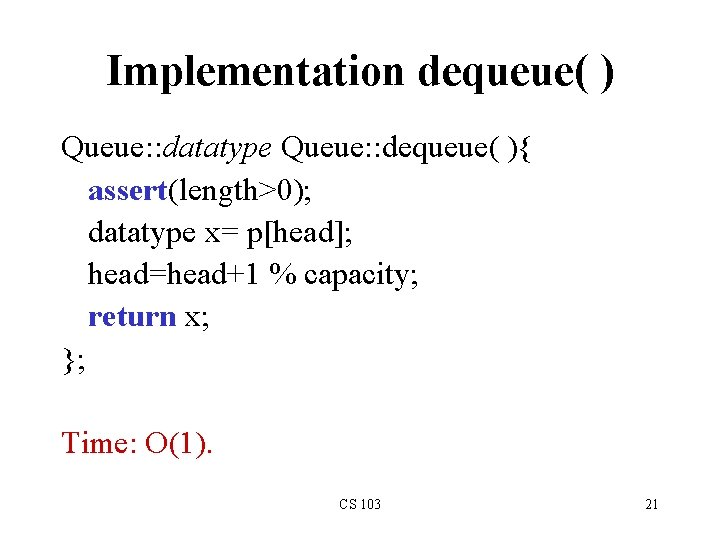Implementation dequeue( ) Queue: : datatype Queue: : dequeue( ){ assert(length>0); datatype x= p[head]; head=head+1 % capacity; return x; }; Time: O(1). CS 103 21